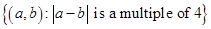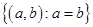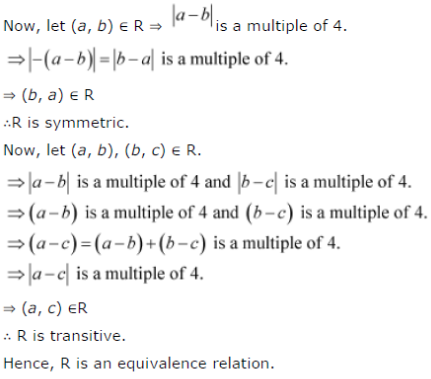# Show that each of the relation R in the set A = { x ∈ Z : 0 ≤ X ≤ 12}, given by:

Show that each of the relation R in the set A = { x ∈ Z : 0 ≤ X ≤ 12} given by:
(i) R =(ii) R =is an equivalence relation. Find the set of all elements related to 1 in each case.